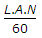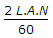# Mechanical Engineering - Hydraulic Machines

### Exercise :: Hydraulic Machines - Section 1

26.

The discharge of a double acting reciprocating pump is (where L = Length of stroke, A = Cross-sectional area of piston, and N = Speed of crank in r.p.m.)

 A. L.A.N B. 2 L.A.N C.D.Explanation:

No answer description available for this question. Let us discuss.

27.

Which of the following turbine is preferred for 0 to 25 m head of water?

 A. Pelton wheel B. Kaplan turbine C. Francis turbine D. none of these

Explanation:

No answer description available for this question. Let us discuss.

28.

The speed ratio of a Francis turbine is defined as the ratio of the theoretical jet velocity to the peripheral speed at inlet.

 A. Yes B. No

Explanation:

No answer description available for this question. Let us discuss.

29.

The overall efficiency of a reaction turbine is the ratio of

 A. power produced by the turbine to the energy actually supplied by the turbine B. actual work available at the turbine to the energy imparted to the wheel C. workdone on the wheel to the energy (or head of water) actually supplied to the turbine D. none of the above

Explanation:

No answer description available for this question. Let us discuss.

30.

Work done by a turbine __________ upon the weight of water flowing per second.

 A. depends B. does not depend